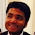Simple Procedure for writing Lewis Structures – Lewis Structures for Nitrogen Oxychloride NOCl | Chemistry Net

# Simple Procedure for writing Lewis Structures – Lewis Structures for Nitrogen Oxychloride NOCl

Lewis structures of NOCl

# Lewis Structures for Nitrogen Oxychloride NOCl

A simple procedure for writing Lewis structures is given in a previous article entitled “Lewis Structures and the Octet Rule”. Several worked examples relevant to this procedure were given in previous posts please see the Sitemap - Table of Contents (Lewis Electron Dot Structures).

Another example  for writing Lewis structures following the above procedure is given below.

Nitrogen Oxychloride is a yellow gas. It is used in synthesis of pharmaceutical products (chlorinated agent).

Step 1:  The central atom will be the N atom since it is the less electronegative. Connect the atoms with single bonds:Step 2: Calculate the # of electrons in π bonds (multiple bonds) using  formula (1) in the article entitled “Lewis Structures and the Octet Rule”.

Where n in this case is 3 since  NOCl consists of three atoms.

Where V = (5 + 6 +7 ) = 18

Therefore, P = 6n + 2 – V = 6 * 3 + 2 – 18 = 2     So,   there are 2π electrons in NOCl .

Step 3 & 4: Therefore, the Lewis electron dot structure of NOCl are as follows:Relevant Posts - Relevant Videos

Lewis Structures|Octet Rule: A Simple Method to write Lewis Structures

Simple Procedure for writing Lewis Structures – Lewis Structures for nitric acid (HNO3)

Lewis Electron - Dot Structure of NO3-

References

1. G.N. Lewis, J.A.C.S, 38, 762-785, (1916)
2. E. C. McGoran, J. Chem. Educ., 68, 19-23 (1991)
3. A.B.P. Lever, J. Chem. Educ., 49, 819-821, (1972)

Key Terms

resonance structures of nitric acid HNO3, Lewis electron structures of nitric acid, chemical formula of HNO3, simple procedure for drawing Lewis structures of nitric acid,

#### 4 comments:

1.2.thank u very much

3.4.this is good